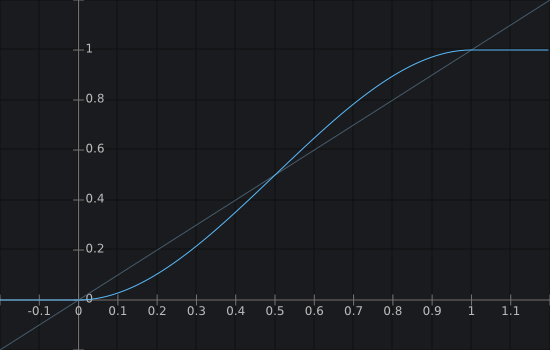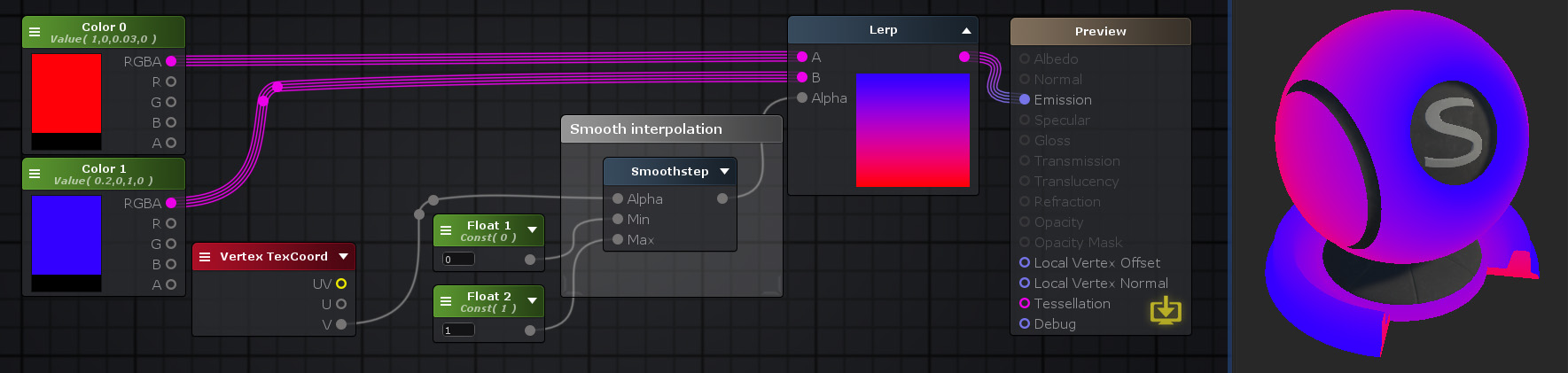## Smoothstep Node

The Smoothstep node calculates a smooth Hermite interpolation value between 0 and 1, if its Input value is in the range [ Min , Max ] range.
Assuming that Max value is greater than Min, a value of 0 is returned if Alpha is less than Min. If on the other way Alpha is greater than Max than a value of 1 is returned.

NOTE 1: Smoothstep between multi-channel data types are done per-channel. If Min and Max have different channel amounts a cast is done to match the one with the most amount of channels.
NOTE 2: Avoid setting an equal value to both Min and Max as it will throw a division by 0 warning.Nodes used: Color, Vertex TexCoord, Float, Smoothstep, Lerp

Node Parameter Description Default Value
Input Specify value to be interpolated. Only visible if the respective input port is not connected. 0
Min Specify minimum reference value to start the interpolation. 0 will be outputted for values of Alpha lower that this.

Only visible if the respective input port is not connected.

0
Max Specify maximum reference value to end the interpolation. 1 will be outputted for values of Alpha higher that this.

Only visible if the respective input port is not connected.

0

Input Port Description Type
Input Specify value to be interpolated. Float 
Min Specify minimum reference value to start the interpolation. 0 will be outputted for values of Alpha lower that this. Float 
Max Specify maximum reference value to end the interpolation. 1 will be outputted for values of Alpha higher that this. Float 

1. ^ Port automatically adapts to all connection types except Matrices and Sampler types.